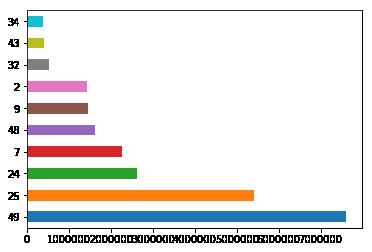﻿ Pandas Excel: Draw a bar plot where each bar will represent one of the top 10 production - w3resource# Pandas Excel: Draw a bar plot, where each bar will represent one of the top 10 production

## Pandas: Excel Exercise-23 with Solution

Write a Pandas program to import given excel data (coalpublic2013.xlsx) into a dataframe and draw a bar plot where each bar will represent one of the top 10 production. Go to Excel data

Sample Solution:

Python Code :

``````import pandas as pd
import numpy as np
import matplotlib.pyplot as plt
plt.show()
``````

Sample Output:Excel Data:

coalpublic2013.xlsx:

Have another way to solve this solution? Contribute your code (and comments) through Disqus.

What is the difficulty level of this exercise?

Test your Programming skills with w3resource's quiz.

﻿

## Python: Tips of the Day

Split a String:

```>>> sentence = 'this is, a python, tutorial, about, idioms.'
>>> sentence.split(', ')
['this is', 'a python', 'tutorial', 'about', 'idioms.']
>>> sentence.split(', ', 2)
['this is', 'a python', 'tutorial, about, idioms.']
>>> sentence.rsplit(', ')
['this is', 'a python', 'tutorial', 'about', 'idioms.']
>>> sentence.rsplit(', ', 2)
['this is, a python, tutorial', 'about', 'idioms.']
```